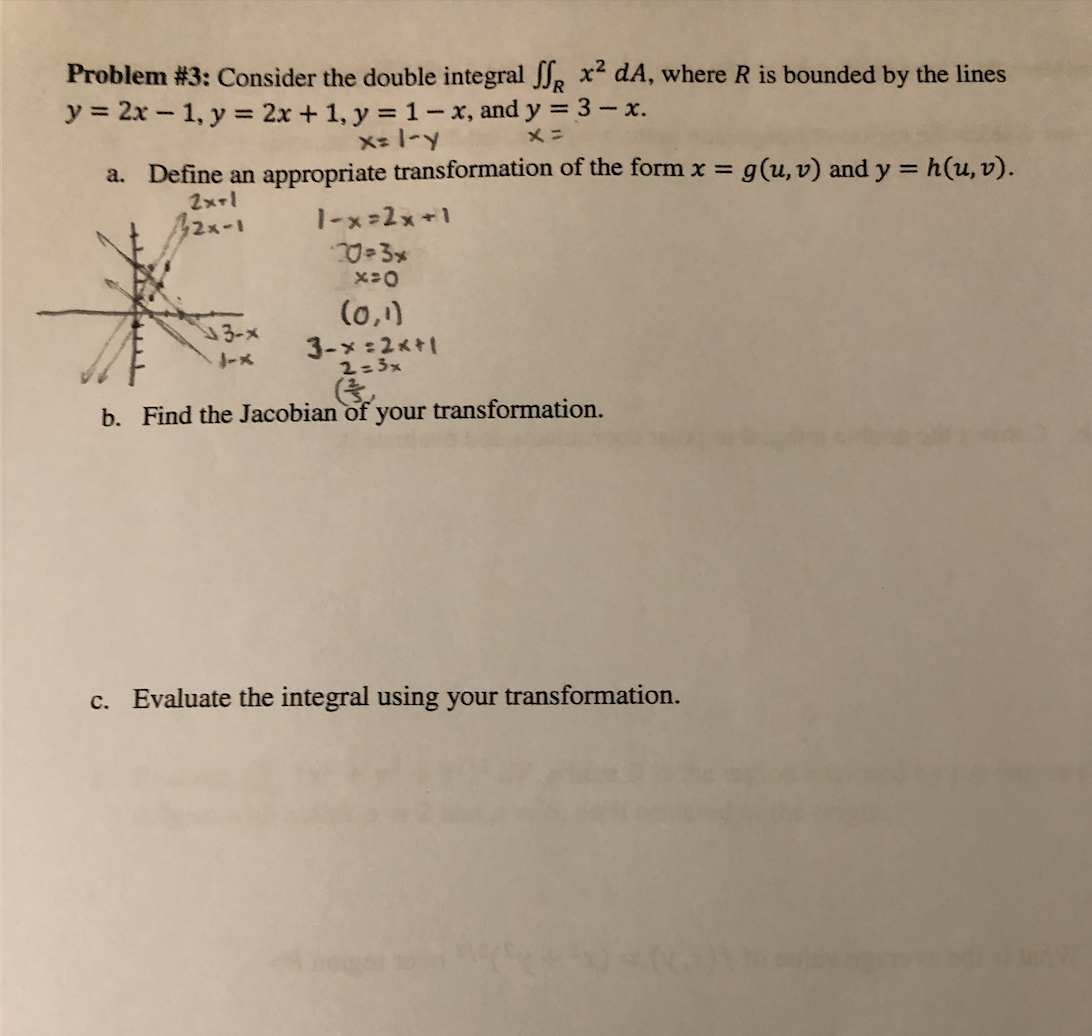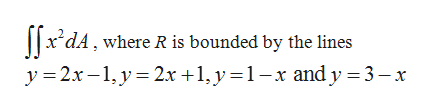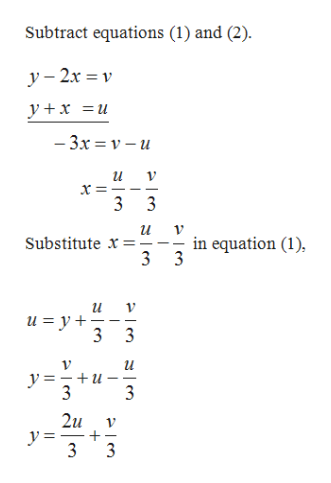# Problem #3: Consider the double integral Jp x dA, where R is bounded by the linesy=2x- 1, y = 2x + 1, y = 1-x, and y 3 - x.X- 1-yメニg(u, v) and yh(u,v).Define an2xrl42x-1appropriate transformation of the form x =a.1-x>2x123(o,)3-x3-x-22-3xb. Find the Jacobian of your transformation.Evaluate the integral using your transformation.c.

Question
3 views

How do I choose  my g(u,v) and h(u,v)?help_outlineImage TranscriptioncloseProblem #3: Consider the double integral Jp x dA, where R is bounded by the lines y=2x- 1, y = 2x + 1, y = 1-x, and y 3 - x. X- 1-y メニ g(u, v) and y h(u,v). Define an 2xrl 42x-1 appropriate transformation of the form x = a. 1-x>2x1 23 (o,) 3-x 3-x-2 2-3x b. Find the Jacobian of your transformation. Evaluate the integral using your transformation. c. fullscreen
check_circle

Step 1

The double integral is given by,help_outlineImage Transcriptionclose|*dА, where R is bounded by the 1lines у 3 2х -1, у%3 2х +1,у%3D1-х and у%33-х fullscreen
Step 2

Now consider,

Step 3

Solve the equations ...help_outlineImage TranscriptioncloseSubtract equations (1) and (2) у - 2х %3D v y x — 3х —D v — и х 3 3 3 in equation (1) 3 3 Substitute -- 3 3 y=-+u 3 3 2u 3 3 fullscreen

### Want to see the full answer?

See Solution

#### Want to see this answer and more?

Solutions are written by subject experts who are available 24/7. Questions are typically answered within 1 hour.*

See Solution
*Response times may vary by subject and question.
Tagged in

### Calculus